# 一个绘制支撑和阻力线的指标实例

### 什么是支撑和阻力线?

• 当线形是水平时，价格在通道中运行，线的角色是建立买入订单以及卖出订单的获利。
• 当线形上升时，价格是上涨趋势，线的角色是建立买入订单。
• 当线形下降时，价格是下跌趋势，线的角色是用于卖出订单的获利。

### 用于在倾斜线中选择第二个点的条件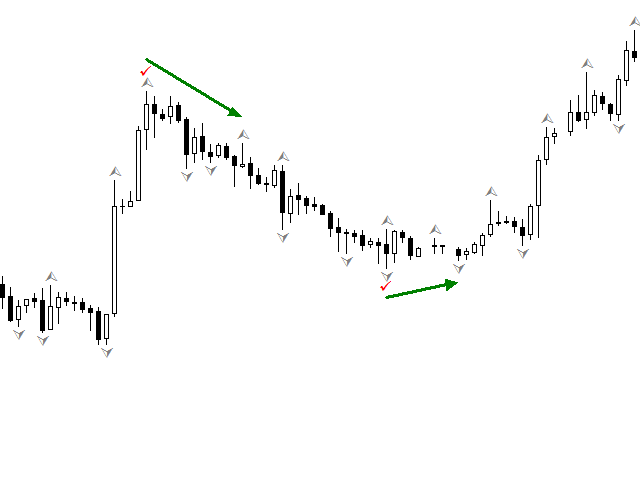### 构建倾斜线指标的实现实例

```   int limit=prev_calculated;
if(limit>3)limit-=3;
if(limit<2)limit=2;

```

```bool up(const double &h[],int q)
{
// 检查一个柱后是否有至少两个柱,
// 如果少于两个柱，这个柱就不可能是分形
// 如果是这样，我们就不做进一步检查而直接返回 false
if(q>=ArraySize(h)-2)return(false);
// 检查前方是否有至少两个柱,
// 如果少于两个柱，这个柱就不可能是分形
// 如果是这样，我们就不做进一步检查而直接返回 false
if(q<2)return(false);
// 检查柱是否为分形，如果是，我们就返回 true
if(h[q]>=h[q-1])
if(h[q]>=h[q-2])
if(h[q]>h[q+1])
if(h[q]>h[q+2])
return(true);
// 如果我们已经进行了全部检查而仍然在这个模块中，这个柱就不是分形，返回 false
return(false);
}
//+------------------------------------------------------------------+
bool down(const double &h[],int q)
{
// 本模块与之前的类似
if(q>=ArraySize(h)-2)return(false);
if(q<2)return(false);
if(h[q]<=h[q-1])
if(h[q]<=h[q-2])
if(h[q]<h[q+1])
if(h[q]<h[q+2])
return(true);
return(false);
}

```

```for(int w=limit;w<rates_total;w++)
{
if(up(high,w))                  //检查柱上是否有分形
{
if(r1==0)r1=w;               //如果它是分形并且我们还没有峰值1, 在此设置峰值1
}
}

```

```if(high[w]>=high[r1])r1=w;                   //与第一个峰值做比较。如果本分形更高，就把该峰值设为第一个
else
{
r2=w;                                    //取得第二个峰值数值
speedr=(high[r2]-high[r1])/(r2-r1);      //计算线形速度
w=r1-1;                                  //返回以绘制新线
}

```

```ResistanceBuffer[w]=high[r1]+speedr*(w-r1);               //为了最小化误差，我们从最顶端开始计算
if(w>r2)                                                  //检查是否有突破
if(high[w]>ResistanceBuffer[w]+Pips_*_Point){r1=0;r2=0;}

```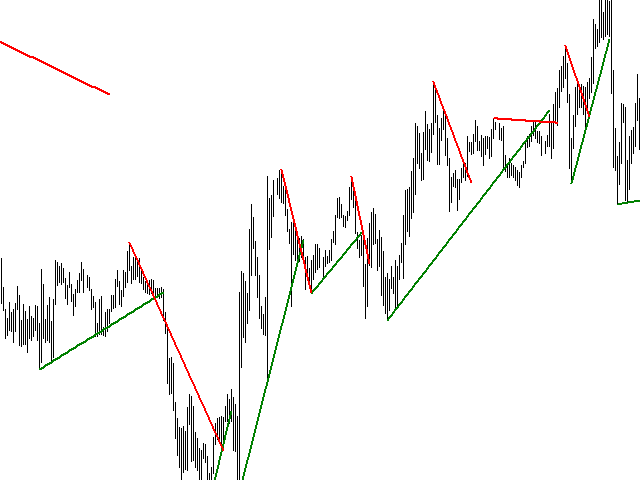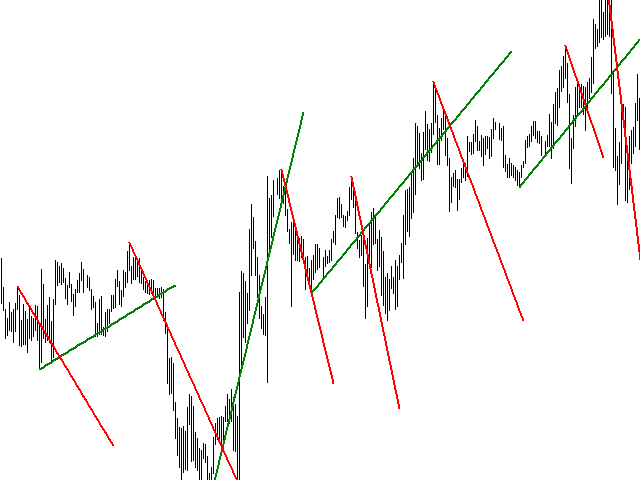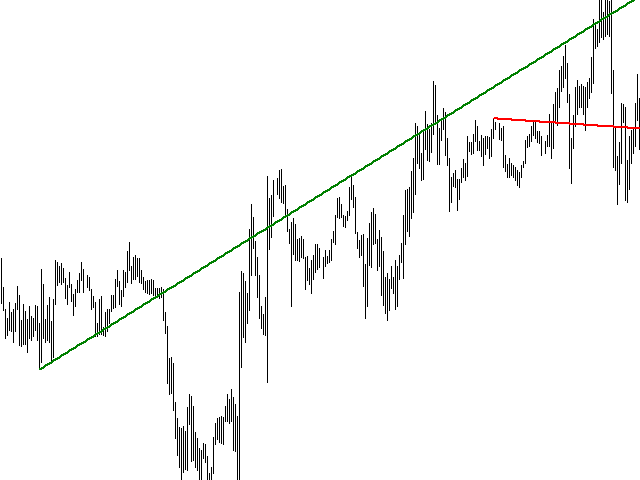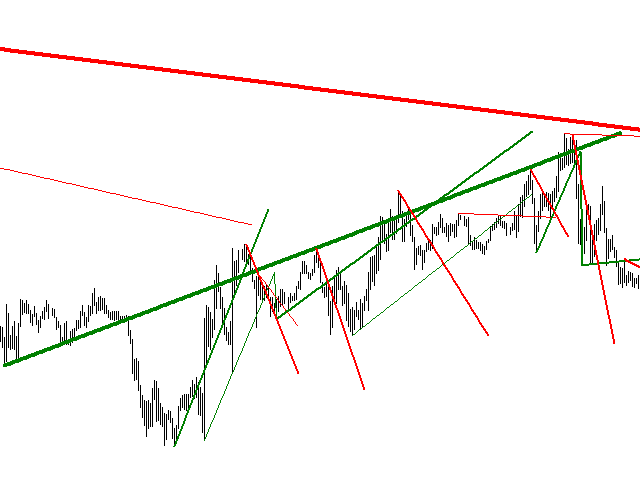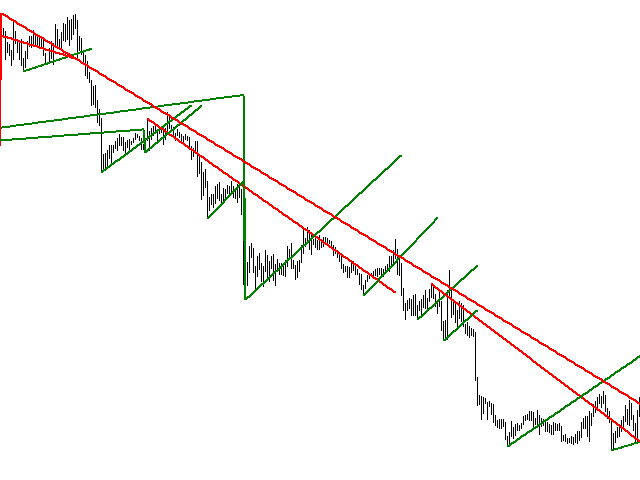### 选择用于绘制水平支撑和阻力水平的点

• 根据前一天(柱)计算的斐波那契水平;
• 根据平均每日 ATR，在前一天平均价格范围上计算的斐波那契水平;
• 在一定范围内最大分形数量;
• 在一定范围内最高价或最低价，开盘价或收盘价的累计;
• 在某一价格上交易量的累计 (市场设置);
• 根据选定模式的价格反转;
• 等等。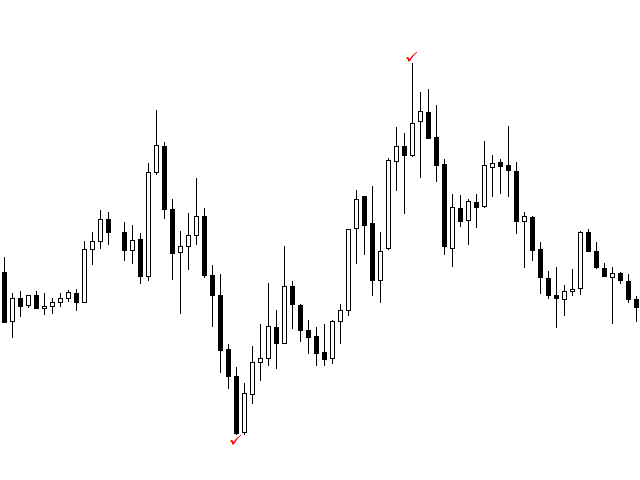### 实现水平支撑和阻力水平的指标实例

```void array_copy(int b_)
{
ArrayResize(time_tf,b_);                         //为我们的数据修改缓冲区大小
ArrayResize(high_tf,b_);
ArrayResize(low_tf,b_);

int total=b_-bars_tf;                            //计算所需复制的数据

CopyTime(_Symbol,Period_TF,0,total,time_tf)      //把缺失的数据复制到数组中
CopyHigh(_Symbol,Period_TF,0,total,high_tf);
CopyLow(_Symbol,Period_TF,0,total,low_tf);

bars_tf=b_;                                      //记住数组大小和数据的数量
}

```

```void f1(double k_fibo,int q,int r,const datetime &time_[],double &b1[],double &b2[])
{
for(int w=q;w<r;w++)
{
int b=f2(time_[w]);         //在更高时段数组中找到当前柱的时间
double h=high_tf[b];        //取得最高价
double l=low_tf[b];         //取得最低价
double hl=h-l;              //取得变化范围
b1[w]=h-hl*k_fibo;          //把计算数值加到支撑缓冲区
b2[w]=l+hl*k_fibo;          //把计算数值加到阻力缓冲区
}
}

```

```int f2(datetime t_)
{
int b_=ArrayBsearch(time_tf,t_);      //在排好序的数组中通过标准搜索来找到柱
if(time_tf[b_]>t_)b_--;               //如果返回了最接近的柱的时间，我们将把它减１
return(MathMax(0,b_-1));              //不要忘记返回柱时要考虑到最小限制
}

```

```   int limit=prev_calculated;
if(limit>0)limit--;

int bar=Bars(_Symbol,Period_TF);
if(bars_tf==0 || bar>bars_tf)array_copy(bar);

f1(0.5,limit,rates_total,time,buffer7,buffer7);           //画出中线
f1(K_Fibo_1,limit,rates_total,time,buffer1,buffer2);      //根据系数１画出水平线
f1(K_Fibo_2,limit,rates_total,time,buffer3,buffer4);      //根据系数2画出水平线
f1(K_Fibo_3,limit,rates_total,time,buffer5,buffer6);      //根据系数3画出水平线

```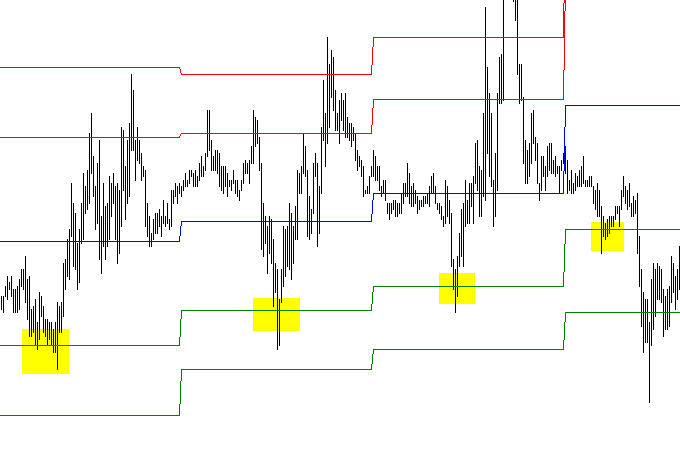### 基于移动平均的支撑和阻力水平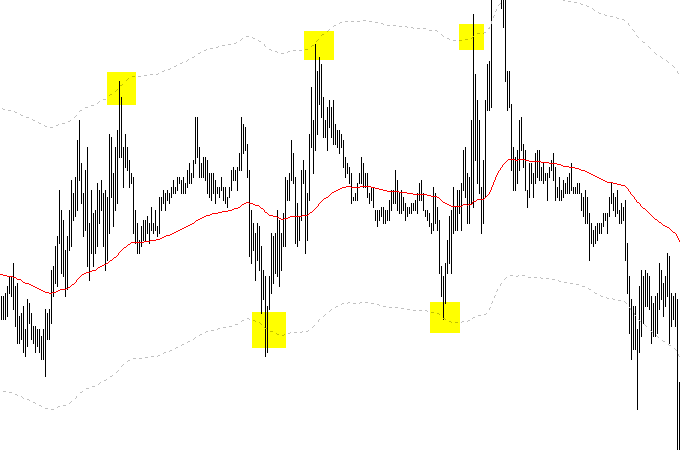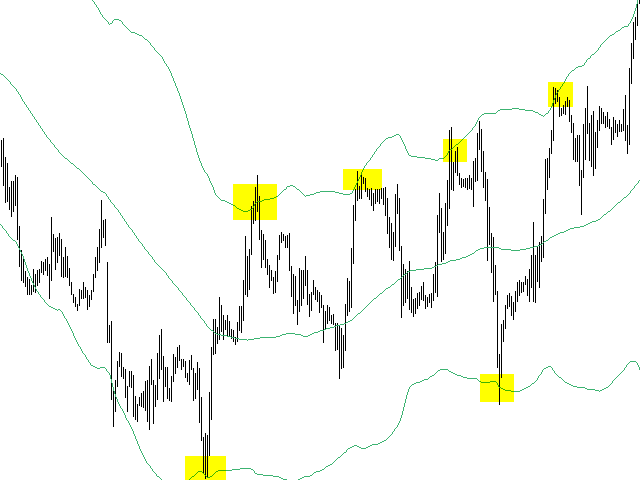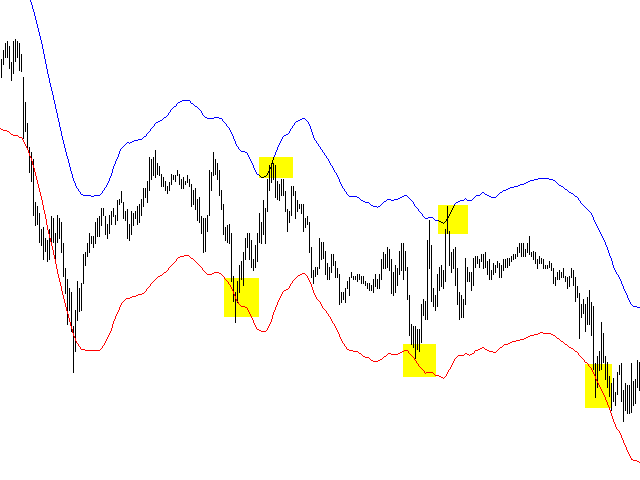## 量化课程

移动端课程